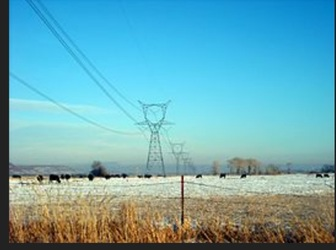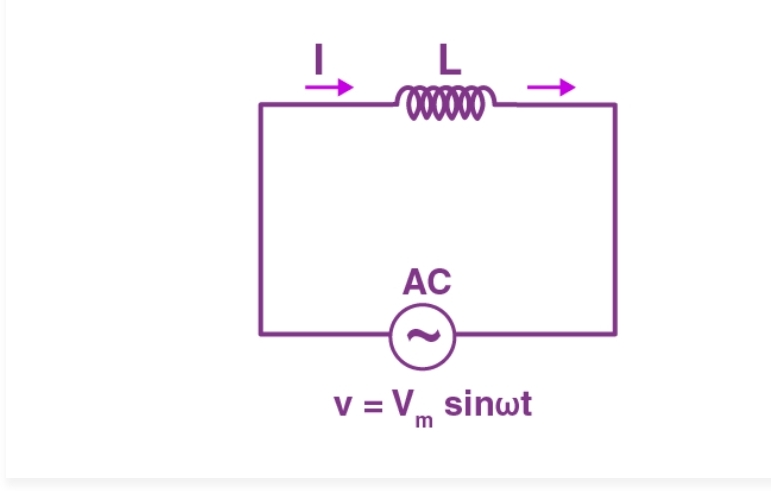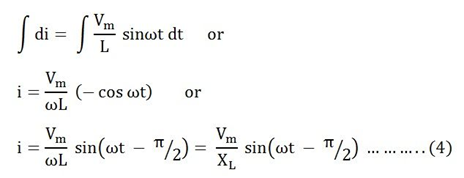# AC Circuit containing Inductance onlyAC Circuit Containing Inductance Only

This type of circuit contains only inductance. There will not be any effect of resistance and capacitance in this circuit. Here, the current will lag behind the voltage by an angle of 90°.

The circuit will be,The inductor will reserves electrical energy in the magnetic field when current flows through it. When this current changes, the time-varying magnetic field causes emf which opposes the flow of current. This opposition to the flow of current is known as inductive reactance.

Let the voltage applied to the circuit be,

v = Vmsinωt ……(1)

The emf induced in the inductor will be,

E = -L x dI / dt

This emf induced in a circuit is equal and opposite to applied voltage.

v = -e ……(2)

Putting the value of e in (2) we get,

v = (-L x dI / dt)

Or

Vmsinωt = L x dI / dt

Or

di = Vm / L sinω dt ……(3)

Integrating both sides, we get,Here,

Inductive reactance is XL = ωL

The value of current will be maximum if sin (ωt – π/2) = 1

Therefore,

Im = Vm / XL ……(5)

Apply the value of Im from (5) and put in (4)

We get,

i = Im sin (ωt – π / 2)

Which implies that the current in pure inductive ac circuits lags the voltage by 900.

This can be expressed in a waveform as,﻿